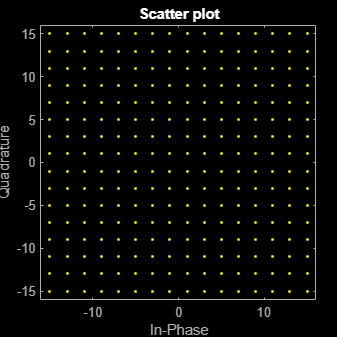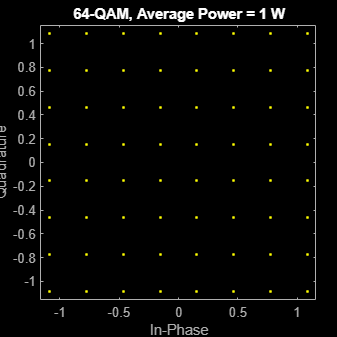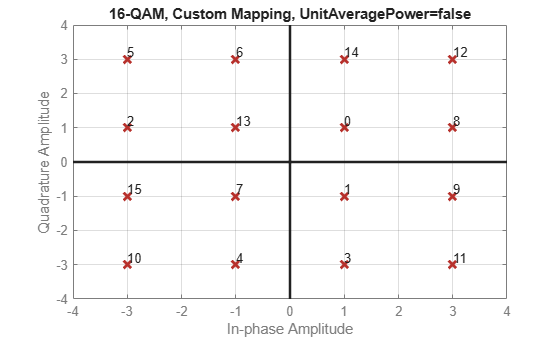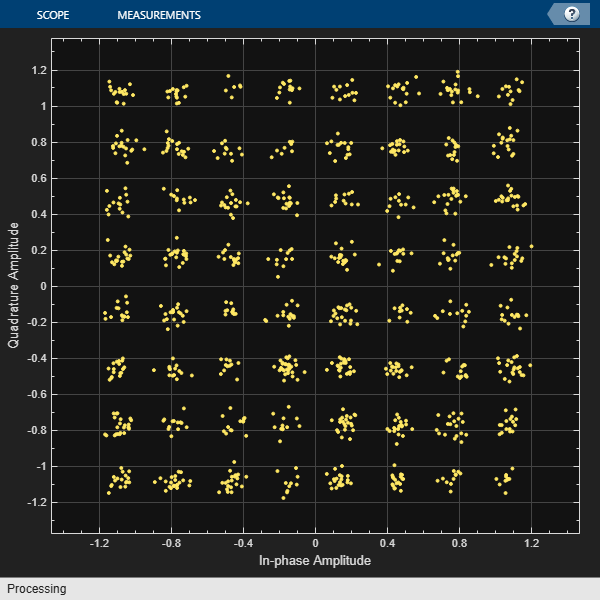qammod

Description

example

y = qammod(x,M) modulates input signal x by using QAM with the specified modulation order M. Output y is the modulated signal.

example

y = qammod(x,M,symOrder) specifies the symbol order.

example

y = qammod(___,Name,Value) specifies options using name-value pair arguments in addition to any of the input argument combinations from previous syntaxes.

Examples

collapse all

Modulate data using QAM and display the result in a scatter plot.

Set the modulation order to 16 and create a data vector containing each of the possible symbols.

M = 16;
x = (0:M-1)';

Modulate the data using the qammod function.

y = qammod(x,M);

Display the modulated signal constellation using the scatterplot function.

scatterplot(y)Set the modulation order to 256, and display the scatter plot of the modulated signal.

M = 256;
x = (0:M-1)';
y = qammod(x,M);
scatterplot(y)Modulate random data symbols using QAM. Normalize the modulator output so that it has an average signal power of 1 W.

Set the modulation order and generate random data.

M = 64;
x = randi([0 M-1],1000,1);

Modulate the data. Use the 'UnitAveragePower' name-value pair to set the output signal to have an average power of 1 W.

y = qammod(x,M,'UnitAveragePower',true);

Confirm that the signal has unit average power.

avgPower = mean(abs(y).^2)
avgPower = 1.0070

Plot the resulting constellation.

scatterplot(y)
title('64-QAM, Average Power = 1 W')Plot QAM constellations for Gray, binary, and custom symbol mappings.

Set the modulation order, and create a data sequence that includes a complete set of symbols for the modulation scheme.

M = 16;
d = [0:M-1];

Modulate the data, and plot its constellation. The default symbol mapping uses Gray ordering. The ordering of the points is not sequential.

y = qammod(d,M,'PlotConstellation',true);Repeat the modulation process with binary symbol mapping. The symbol mapping follows a natural binary order and is sequential.

z = qammod(d,M,'bin','PlotConstellation',true);Create a custom symbol mapping.

smap = randperm(M)-1;

Modulate and plot the constellation.

w = qammod(d,M,smap,'PlotConstellation',true);Modulate a sequence of bits using 64-QAM. Pass the signal through a noisy channel. Display the resultant constellation diagram.

Set the modulation order, and determine the number of bits per symbol.

M = 64;
k = log2(M);

Create a binary data sequence. When using binary inputs, the number of rows in the input must be an integer multiple of the number of bits per symbol.

data = randi([0 1],1000*k,1);

Modulate the signal using bit inputs, and set it to have unit average power.

txSig = qammod(data,M,'InputType','bit','UnitAveragePower',true);

Pass the signal through a noisy channel.

rxSig = awgn(txSig,25);

Plot the constellation diagram.

cd = comm.ConstellationDiagram('ShowReferenceConstellation',false);
cd(rxSig)Demodulate a fixed-point QAM signal and verify that the data is recovered correctly.

Set the modulation order as 64, and determine the number of bits per symbol.

M = 64;
bitsPerSym = log2(M);

Generate random bits. When operating in bit mode, the length of the input data must be an integer multiple of the number of bits per symbol.

x = randi([0 1],10*bitsPerSym,1);

Modulate the input data using a binary symbol mapping. Set the modulator to output fixed-point data. The numeric data type is signed with a 16-bit word length and a 10-bit fraction length.

y = qammod(x,M,'bin','InputType','bit','OutputDataType', ...
numerictype(1,16,10));

Demodulate the 64-QAM signal. Verify that the demodulated data matches the input data.

z = qamdemod(y,M,'bin','OutputType','bit');
s = isequal(x,double(z))
s = logical
1

Input Arguments

collapse all

Input signal, specified as a scalar, vector, matrix, or 3-D array. The elements of x must be binary values or integers that range from 0 to (M – 1), where M is the modulation order.

Note

To process input signal as binary elements, set the 'InputType' name-value pair to 'bit'. For binary inputs, the number of rows must be an integer multiple of log2(M). Groups of log2(M) bits are mapped onto a symbol, with the first bit representing the MSB and the last bit representing the LSB.

Data Types: double | single | fi | int8 | int16 | uint8 | uint16

Modulation order, specified as a power-of-two scalar integer. The modulation order specifies the number of points in the signal constellation.

Example: 16

Data Types: double

Symbol order, specified as 'gray', 'bin', or a vector.

• 'gray' — Use Gray Code ordering

• 'bin' — Use natural binary-coded ordering

• Vector — Use custom symbol ordering

Vectors must use unique elements whose values range from 0 to M – 1. The first element corresponds to the upper-left point of the constellation, with subsequent elements running down column-wise from left to right.

Example: [0 3 1 2]

Data Types: char | double

Name-Value Arguments

Specify optional comma-separated pairs of Name,Value arguments. Name is the argument name and Value is the corresponding value. Name must appear inside quotes. You can specify several name and value pair arguments in any order as Name1,Value1,...,NameN,ValueN.

Example: y = qammod(x,M,symOrder,'InputType','bit')

Input type, specified as the comma-separated pair consisting of 'InputType' and either 'integer' or 'bit'. If you specify 'integer', the input signal must consist of integers from 0 to M – 1. If you specify 'bit', the input signal must contain binary values, and the number of rows must be an integer multiple of log2(M).

Data Types: char

Unit average power flag, specified as the comma-separated pair consisting of 'UnitAveragePower' and a numeric or logical 0 (false) or 1 (true). When this flag is 1 (true), the function scales the constellation to the average power of one watt referenced to 1 ohm. When this flag is 0 (false), the function scales the constellation so that the QAM constellation points are separated by a minimum distance of two.

Output data type, specified as the comma-separated pair consisting of 'OutputDataType' and a numerictype object.

For more information on constructing these objects, see numerictype (Fixed-Point Designer). If you do not specify 'OutputDataType', data type is double if the input is of data type double or built-in integer and single if the input is of data type single.

Option to plot constellation, specified as the comma-separated pair consisting of 'PlotConstellation' and a numeric or logical 0 (false) or 1 (true) To plot the QAM constellation, set 'PlotConstellation' to true.

Output Arguments

collapse all

Modulated signal, returned as a complex scalar, vector, matrix, or 3-D array of numeric values. For integer inputs, output y has the same dimensions as input signal x. For bit inputs, the number of rows in y is the number of rows in x divided by log2(M).

Data Types: double | single

collapse all

Gray Code

A Gray code, also known as a reflected binary code, is a system where the bit patterns in adjacent constellation points differ by only one bit.

Compatibility Considerations

expand all

Errors starting in R2018b